# How to generate random numbers in Excel without duplicates

In this article, we'll discuss a few different formulas to randomize in Excel without repeating numbers. Also, we will show you a universal Random Generator that can produce a list of random numbers, dates, and strings with no repeats.

As you probably know, Microsoft Excel has several functions for generating random numbers such as RAND, RANDBETWEEN and RANDARRAY. However, there is no guarantee that the result of any function will be duplicate free.

This tutorial explains a few formulas for creating a list of unique random numbers. Please pay attention that some formulas only work in the latest version of Excel 365 and 2021 while others can be used in any version of Excel 2019, Excel 2016, Excel 2013 and earlier.

## Get a list of unique random numbers with predefined step

Only works in Excel 365 and Excel 2021 that support dynamic arrays.

If you have the latest Excel version, the easiest way for you to get a list of unique random numbers is to combine 3 new dynamic array functions: SORTBY, SEQUENCE and RANDARRAY:

SORTBY(SEQUENCE(n), RANDARRAY(n))

Where n is the number of random values you want to get.

For example, to create a list of 5 random numbers, use 5 for n:

`=SORTBY(SEQUENCE(5), RANDARRAY(5))`

Enter the formula in the topmost cell, press the Enter key, and the results will spill automatically over the specified number of cells.

As you can see in the screenshot below, this formula actually sorts numbers from 1 to 5 in random order. If you need a classic random number generator with no repeats, then please check out other examples that follow below.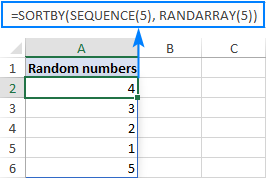In the above formula, you only define how many rows to fill. All other arguments are left to their default values, meaning the list will start at 1 and will be incremented by 1. If you'd like a different first number and increment, then set your own values for the 3rd (start) and 4th (step) arguments of the SEQUENCE function.

For instance, to start at 100 and increment by 10, use this formula:

`=SORTBY(SEQUENCE(5, , 100, 10), RANDARRAY(5))`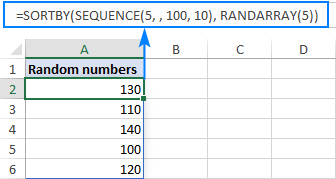How this formula works:

Working from the inside out, here's what the formula does:

• The SEQUENCE function creates an array of sequential numbers based on the specified or default start value and incrementing step size. This sequence goes to the array argument of SORTBY.
• The RANDARRAY function creates an array of random numbers of the same size as the sequence (5 rows, 1 column in our case). The min and max value do not really matter, so we can leave these to defaults. This array goes to the by_array argument of SORTBY.
• The SORTBY function sorts the sequential numbers generated by SEQUENCE using an array of random numbers produced by RANDARRAY.

Please keep in mind that this simple formula creates a list of non repeating random numbers with a predefined step. To bypass this limitation, use an advanced version of the formula described below.

## Generate a list of random numbers with no duplicates

Only works in Excel 365 and Excel 2021 that support dynamic arrays.

To generate random numbers in Excel without duplicates, use one of the below generic formulas.

Random integers:

INDEX(UNIQUE(RANDARRAY(n^2, 1, min, max, TRUE)), SEQUENCE(n))

Random decimals:

INDEX(UNIQUE(RANDARRAY(n^2, 1, min, max, FALSE)), SEQUENCE(n))

Where:

• N is the number of values to generate.
• Min is the minimal value.
• Max is the maximum value.

For example, to create a list of 5 random integers from 1 to 100 with no repeats, use this formula:

`=INDEX(UNIQUE(RANDARRAY(5^2, 1, 1, 100, TRUE)), SEQUENCE(5))`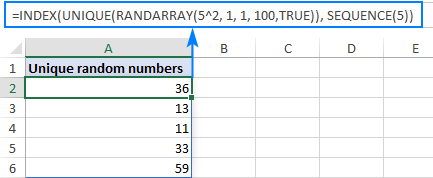To generate 5 unique random decimal numbers, put FALSE in the last argument of RANDARRAY or omit this argument:

`=INDEX(UNIQUE(RANDARRAY(5^2, 1, 1, 100)), SEQUENCE(5))`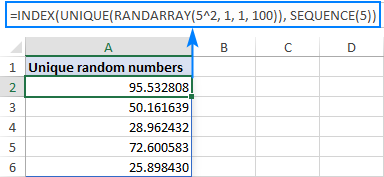How this formula works:

At first sight the formula may look a bit tricky, but upon a closer look its logic is very straightforward:

• The RANDARRAY function creates an array of random numbers based on the min and max values that you specify. To determine how many values to generate, you raise the desired number of uniques to the power of 2. Because the resulting array may have no one knows how many duplicates, you need to provide a sufficient array of values for UNIQUE to choose from. In this example, we need only 5 unique random numbers but we instruct RANDARRAY to produce 25 (5^2).
• The UNIQUE function removes all duplicates and "feeds" a duplicate-free array to INDEX.
• From the array passed by UNIQUE, the INDEX function extracts the first n values as specified by SEQUENCE (5 numbers in our case). Because values are already in random order, it does not really matter which ones survive.

Note. On very large arrays, this formula may be a bit slow. For example, to get a list of 1,000 unique numbers as the final result, RANDARRAY would have to generate of array of 1,000,000 random numbers (1000^2) internally. In such situations, instead of raising to power, you can multiply n by, say, 10 or 20. Just keep in mind please that the smaller array is passed to the UNIQUE function (small relative to the desired number of unique random values), the bigger the chance that not all cells in the spill range will be filled with the results.

## Create a range of non-repeating random numbers in Excel

Only works in Excel 365 and Excel 2021 that support dynamic arrays.

To generate a range of random numbers with no repeats, you can use this formula:

INDEX(UNIQUE(RANDARRAY(n^2, 1, min, max)), SEQUENCE(rows, columns))

Where:

• n is the number of cells to fill. To avoid manual calculations, you can supply it as (no. of rows * no. of columns). For example, to fill 10 rows and 5 columns, use 50^2 or (10*5)^2.
• Rows is the number of rows to fill.
• Columns is the number of columns to fill.
• Min is the lowest value.
• Max is the highest value.

As you may notice, the formula is basically the same as in the previous example. The only difference is the SEQUENCE function, which in this case defines both the number of rows and columns.

For instance, to fill a range of 10 rows and 3 columns with unique random numbers from 1 to 100, use this formula:

`=INDEX(UNIQUE(RANDARRAY(30^2, 1, 1, 100)), SEQUENCE(10, 3))`

And it will produce an array of random decimals without repeating numbers: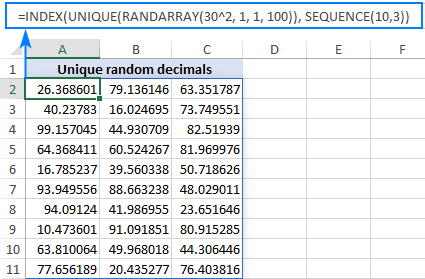If you need whole numbers, then set the last argument of RANDARRAY to TRUE:

`=INDEX(UNIQUE(RANDARRAY(30^2, 1, 1, 100, TRUE)), SEQUENCE(10,3))`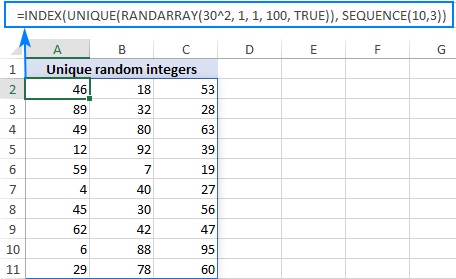## How to generate unique random numbers in Excel 2019, 2016 and earlier

As no version other than Excel 365 and 2021 supports dynamic arrays, none of the above solutions work in earlier versions of Excel. However, this does not mean there is no solution at all, you'll just have to perform a few more steps:

1. Create a list of random numbers. Based on your needs, use either:
• The RAND function to generate random decimals between 0 and 1, or
• The RANDBETWEEN function to produce random integers in the range that you specify.

Be sure to generate more values than you actually need because some will be duplicates and you'll delete them later.

For this example, we are creating a list of 10 random integers between 1 and 20 by using the below formula:

`=RANDBETWEEN(1,20)`

To enter the formula in multiple cells in one go, select all the cells (A2:A15 in our example), type the formula in the formula bar and press Ctrl + Enter. Or you can enter the formula in the first cell as usual, and then drag it down to as many cells as needed.

Anyway, the result will look something like this: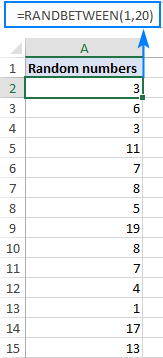As you may notice, we've entered the formula in 14 cells, though eventually we only need 10 unique random numbers.

2. Change formulas to values. As both RAND and RANDBETWEEN recalculate with every change on the worksheet, your list of random numbers will be continuously changing. To prevent this from happening, use Paste Special > Values to convert formulas to values as explained in How to stop random numbers from recalculating.

To make sure you've done it right, select any number and look at the formula bar. It should now display a value, not a formula: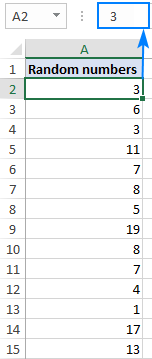3. Delete duplicates. To have it done, select all the numbers, go to the Data tab > Data tools group, and click Remove Duplicates. In the Remove Duplicates dialog box that appears, simply click OK without changing anything. For the detailed steps, please see How to remove duplicates in Excel.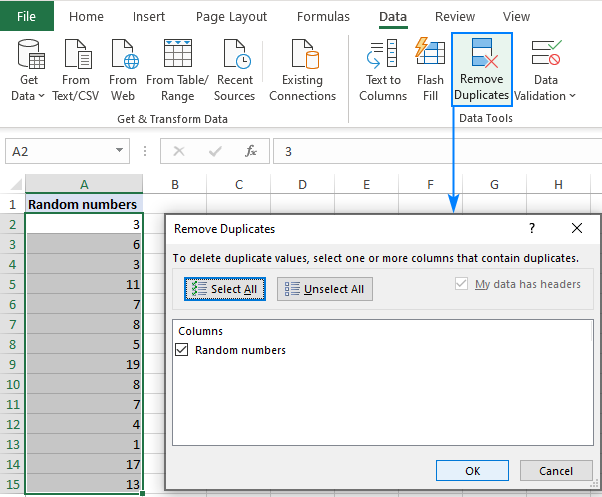Done! All duplicates are gone, and you can now delete the excess numbers.

Tip. Instead of Excel's built-in tool, you can use our advanced Duplicate Remover for Excel.

## How to stop random numbers from changing

All of the randomizing functions in Excel including RAND, RANDBETWEEN and RANDARRAY are volatile, meaning they recalculate every time the spreadsheet is changed. As the result, new random values are produced with every change. To prevent generating new numbers automatically, use the Paste Special > Values feature to replace formulas with static values. Here's how:

1. Select all the cells with your random formula and press Ctrl + C to copy them.
2. Right click the selected range and click Paste Special > Values. Alternatively, you can press Shift + F10 and then V, which is the shortcut for this option.

For the detailed steps, please see How to change formulas to values in Excel.

## Random number generator for Excel with no repeats

The users of our Ultimate Suite do not really need any of the above solutions because they already have a universal Random Generator in their Excel. This tool can easily produce a list of non-repeating integers, decimals numbers, dates, and unique passwords. Here's how:

1. On the Ablebits Tools tab, click Randomize > Random Generator.
2. Select the range to fill with random numbers.
3. On the Random Generator pane, do the following:
• Choose the desired value type: integer, real number, date, Boolean, custom list, or string (ideal for generating strong unique passwords!).
• Set up the From and To values.
• Select the Unique values check box.
• Click Generate.

That's it! The selected range gets filled with non-repeating random numbers at once: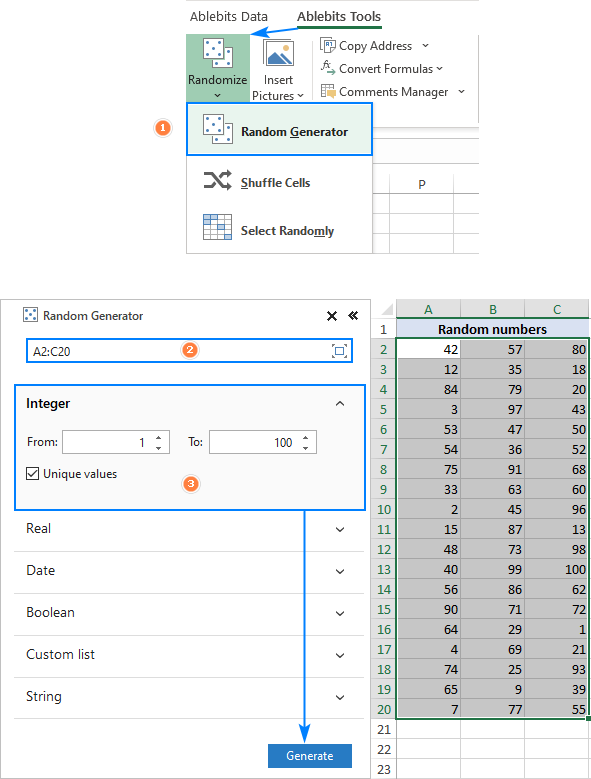If you are curious to try this tool and explore other fascinating features included with our Ultimate Suite, you are welcome to download a trial version.

That's how to randomize numbers in Excel without duplicates. I thank you for reading and hope to see you on our blog next week!

Generate unique random numbers in Excel (.xlsx file)

## You may also be interested in

1. Hi there,

I have tried to apply this formula =INDEX(UNIQUE(RANDARRAY(120^2, 1, 1, 50, TRUE)), SEQUENCE(15,8)) to get 15 rows * 8 columns of numbers 1 to 50 with no repeats in the rows. The formula works for like 5 or 6 rows correctly and then the #REF! error populates the other cells down.

Also, would it be possible to wrap this formula with a sortby function such that instead of the result reading as (eg. 38,15,16,4,12,46,43,48), I get the same values but sorted like this (4,12,15,16,38,43,46,48)

• Hi! You want to write 50 numbers in 120 cells without repeating them? Sorry, that is not possible. Increase the quantity of numbers. For example,

=INDEX(UNIQUE(RANDARRAY(120^2, 1, 1, 500, TRUE)), SEQUENCE(15,8))

• Wow, that was quick. Thanks Alexander.

My apologies. Here is a better example of what happens
S.No.
1 - 11,18,24,27,29,40,42,50
2 - 1,6,8,19,23,26,46,47
3 - 3,9,13,14,25,28,34,39
4 - 4,5,10,20,32,35,37,41
5 - 7,12,16,30,33,36,43,45
6 - 2,15,17,22,38,44,48,49
7 - 21,31,#REF!,#REF!,#REF!,#REF!,#REF!,#REF!
8 - #REF!,#REF!,#REF!,#REF!,#REF!,#REF!,#REF!,#REF!
9 - #REF!,#REF!,#REF!,#REF!,#REF!,#REF!,#REF!,#REF!

• Did you use the formula I gave you?

• i am in need of values from 1 to 50. replacing 50 with 500 does remove the #Ref! errors but does not help as the numbers are above 50.

• Then find a way to place 50 numbers in 120 cells without repeating them. I think this will be very much appreciated.

2. That's perfect!
Thank you very much, Svetlana!

3. Hi all,

I 'm using VBA with randombetween function and I think it is the best way to generate unique random numbers (as example range of random numbers between 100 to 1000) .
The VBA code check the current generated number with all previous numbers if exist then it must be regenerated again and so on.

Regards

4. I have a random generator file for choosing swabbing sites. Once a year we need to go through all the sites, and we swab weekly. How can I format the file so that it does not ever duplicate a swab site for the year? Is this possible? We are randomly picking 7 sites weekly, but I don't want it to duplicate till all the sites have been swabbed, and then it could start over.

The equation I'm currently using is: =INDEX(UNIQUE(RANDARRAY(7^2,1,1,312,TRUE)),SEQUENCE(7))

I could randomize all 312 sites, just wondering if there is a better way to accomplish the task. Multiple people use the file, so I want something user friendly for everyone using it.

Is this possible to do in Excel?

• Hello!
Random values can only be generated over a continuous range of numbers. You can check the site check box in a separate column. Then you create a new list of unverified sites. Change the number 312 in the formula to a smaller one.

5. Hi There- I Loved these formulas!! It was super helpful, though I am trying to see if there is a related formula (or pair of them) for allowing 'less than e.g. 10% repeat rates. For example, if we started with 1500 product testers, and we had 100 testers per product, how could we generate groups of 100 per product such that no more than 10% of the 100 were repeats (i.e. groups of 100 were 90% unique). Thanks in advance for your insight! Best Regards, JF

• Hello!
Unfortunately, Excel formulas cannot control how many duplicates will appear in the list.

6. Hello! I am using this formula but need two columns of random numbers. 1-9 are the numbers. If first column number is 1-5 then second column needs to be greater than 5 and vica versa, if first column is >5 then second column 5 =<.
I was thinking I’d have to imbed another formula into a if then? I’m pretty rusty with excel.

• Hello!
Sorry, I do not fully understand the task. What formula are you using? Write an example of the result you want to get.

• Thanks for the reply! So I’m assigning daily schedules to 9 employees for Morning and afternoon. 9 schedules, 9 employees. I used
=sortby(sequence(9),randarray(9)) to assign the AM schedules. For PM, anyone with a schedule 1-5 in AM should be assigned schedules 6-9 and if 6-9 in am, 1-5 in afternoon.
I did a combination of IF and randbetween but of course get duplicates in afternoon

• So far I need this to not have duplicates:
=if(b12<=5,randbetween(6,9),randbetween1,4))

• Hi!
Write an example of what values you want to see in column B and column C.
I think that for 5 people (1-5) it is impossible to assign 4 numbers (6-9) without duplicates! Or have I misunderstood something?

• B. C
5. 7
3. 6
7. 4
4. 5
8. 1
6. 2
2. 3
1. 9

Hopefully formatting will hold when I post this.

• Correction. That 9 in C column can be an 8. This example has 8 employees and 8 schedules. Different days have different numbers of employees available for schedules.

• Hi!
As far as I can see from your last comment, your task is now different from the original one. Your example result is completely different from what you wrote earlier. In these numbers, I do not see any pattern. I don’t think I can help with such a task.

7. I am also having a problem with the formula:

=INDEX(UNIQUE(RANDARRAY(4, 10, 1, 10, TRUE)), SEQUENCE(4), {1,2,3,4,5,6,7,8,9,10}).

I am basically trying to produce a Sudoku table much like the example above with the tennis players.

I want to have 4 inspectors test 10 parts with them testing at the same time (if part 1 is being tested by inspector 1, it cannot be available to the other 3 inspectors during that run and inspector 1 should only be shown part 1 one time).

The array should only produce 1 number across a row and column.

• Hi!
The information you provided is not enough to understand your case and give you any advice, sorry. Describe in detail what problem you have, and I will try to help you.

8. thanks for your blog. I have volunteered to build a tennis schedule for my club, this requires random teams of 4 for each of 4 time slot (total 16 per week). Unfortunately there are 20 players, so 4 'sit out' each week. E.G. week 1, players 1-16 play, (these are to be randomized) and players 17-20 'sit out'. In week 2 perhaps players 5-20 play and 1-4 'sit out'. Each number has a name associated with it.
I tried this formula across my players both in and out =INDEX(UNIQUE(RANDARRAY(30^2,1,1,20,TRUE)),SEQUENCE(1,20))
but the 'out' players were treated like any other group of 4 and so they were not fairly randomized (they should only sit out 2 times in an 8 week rotation.
I was wondering if there is a way to input a dynamic range so that each week it can look at my non randomized list of players who are out for a week and generate the random numbers from the residual list of 1-20 for my list of players :)

• Any update to Richard's challenge up above? I'm in the same boat having volunteered to build a Pickleball schedule for my club and facing the same challenge

• Hi!
Your request goes beyond the advice we provide on this blog. This is a complex solution that cannot be found with a single formula. If you have a specific question about the operation of a function or formula, I will try to answer it.

9. Hello,
thanks for this interesting topic.
I wondered to know how to generate unrepeated random number starting always by the same number, like 12 for exemple?

• Hi,
The formula below will do the trick for you:

=--("12"&RANDBETWEEN(100,200))

EXISTE UMA FORMULA EM EXCEL PARA GERAR NUMEROS ALEOTÓRIOS EXCLUINDO ALGUNS.
EX GERA RANDOM ENTRE 1 E 30 EXCLUINDO OS NUMEROS 11,24 E 29

11. Hi, I have a query regarding the following function:

=INDEX(UNIQUE(RANDARRAY(20, 3, 1, 100, TRUE)), SEQUENCE(10), {1,2,3})

You have provided this formula under the heading "Create a range of non-repeating random numbers in Excel". But the example array you have shown contains repeating numbers. Eg: 65 appears twice, 25 apears twice, 61 appears twice and so on. Your array does not appear to contain 30 unique random numbers, because several of them are repeated.

I am trying to create a 5 x 5 array, with randomly generated numbers between 1 and 50, without any duplicates. So the result I need is 25 unique numbers in the range 1 to 50, in a 5 x 5 table. This is my formula based on your example:

=INDEX(UNIQUE(RANDARRAY(50, 5, 1, 50, TRUE)), SEQUENCE(5), {1,2,3,4,5})

But I am yet to get a 5 x 5 array with 25 unique numbers. There are always several numbers that are repeated in the array, sometimes in duplicate, sometimes even in triplicate. I thought at first, maybe my range (1 to 50) is not big enough? So I extended it from 1 to 100, but still got repeating numbers.

In any case, I thought the UNIQUE function would weed out any duplicated numbers. So really, it shouldn't matter whether I have 1 to 50 or even 1 to 500 as my range, isn't the job of "UNIQUE" to stop two numbers that are the same from ending up in the array?

My question is: how can I get my 5 x 5 array to have 25 unique numbers with values between 1 and 50 without any duplication of numbers?

I am using the latest 365 version of Excel.

Thank you for any assistance you can offer.

• Hi Shani,

The formula is incorrect, sorry for misleading you. Trying it figure it out now (and how I could have overlooked duplicate numbers in the screen). We will post an update asap. Again, my apologies for the inconvenience.

• Shani,

We have fixed the formula and updated the example (thanks to Alex, one of our best Excel gurus, for this solution!).

During my tests, the improved formula worked perfect with no repeats. We will appreciate if you could test it on your side too and let us know about your results. Thank you in advance!

12. In my question/comment, I meant to say that I'm interested in generating unique random numbers across rather than down.

• Dear Dr. Rapoport,

If you use Excel 365, then you can find a solution in How to create a range of unique random numbers. That formula produces no duplicates in either rows or columns.

13. Generate random numbers without Ablebits works down columns. It does not seem to work across rows, because if I produce an array using any of these methods, there are often duplicates in a row. Is there a way to fix that? Thanks.

• Hello!
There are a lot of formulas in the article. Clarify which of them are you talking about?
Try this formula

=INDEX(UNIQUE(RANDARRAY(2, 10, 1, 100)), SEQUENCE(1), {1,2,3,4,5,6,7,8,9,10})

Thank You.